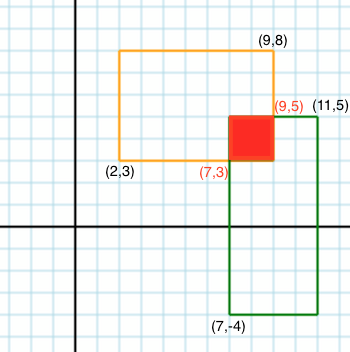## Finding the Overlap of Two Rectangles in Ruby

In our 3rd-to-last week at General Assembly, we spent an entire week learning about Computer Science basics including sorting algorithms, tree structures, and how to solve puzzles with code.

In the process of studying this material, I came across the following puzzle: Create a function that prints out the overlap of two rectangles based on two diagonal coordinates of each rectangle.

Input:

``````\$ square = [[2,3],[9,8],[7,-4],[11,5]]
\$ check_square(square)
``````

Expected output:

``````\$ [[7, 3], [9, 5]]
``````

Or for those who are visually inclined:To solve this problem, we need to first check the coordinates provided to ensure that they both represent the bottom left and top right corners of each rectangle. To do so, we check if the x value from the first coordinate (2 in this case) is larger than the x value from the second coordinate (9 in this case). If it’s larger, the coordinates represent the top left and bottom right coordinates (not good!) so we need to switch those coordinates. The same goes for the y value of each coordinate.

Once the coordinates are fixed, we call bound_box() which determines the overlap. To do so, we first find the max x and y values from the bottom left corners of each rectangle. Then we find the min x and y values from the top right corners of each rectangle. The result is the coordinates for the overlap rectangle.

Here’s my solution: